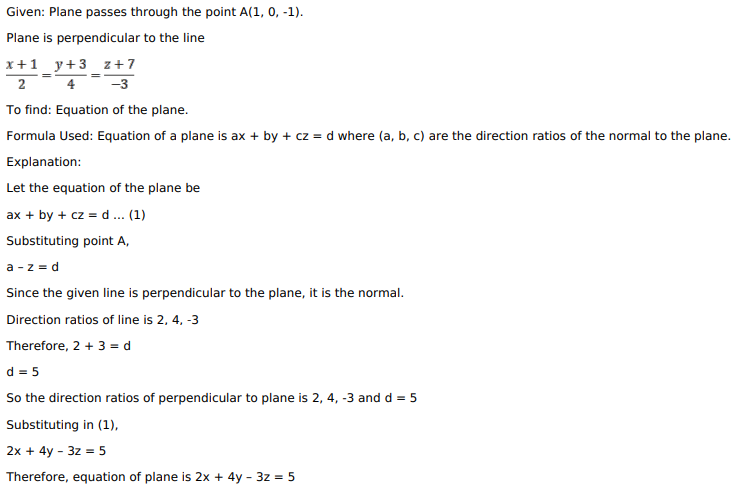# Mark against the correct answer in each of the following:Question:

Mark against the correct answer in each of the following:

The equation of a plane through the point $A(1,0,-1)$ and perpendicular to the line $\frac{x+1}{2}=\frac{y+3}{4}=\frac{z+7}{-3}$ is

A. $2 x+4 y-3 z=3$

B. $2 x-4 y+3 z=5$

C. $2 x+4 y-3 z=5$

D. $x+3 y+7 z=-6$

Solution: